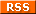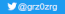# Tiny bitfield based text renderer

### Introduction

I have been busy doing tiny text rasterizer for a 256 bytes Linux intro which use the Linux framebuffer, the idea is to draw some 1-bit letters (three big ones in my case; a logo) with the smallest amount of x86 code possible.

An easy way would be to use the framebuffer console (fbcon) but i also needed to apply some post-process to the letters once they are drawn thus the frame buffer device (/dev/fb*) was more adequate (fbcon draw on top of /dev/fb*), the frame buffer device is quite low-level, just giving you the minimum to manipulate graphics (no direct way to draw rectangles etc.) so a standalone text renderer had to be done.

I first wrote specific x86 code which resulted in about 69 bytes to draw a big three letters string "ORZ" with a custom tiny typeface (just lines and no anti aliasing), this does not use bit field but some fun x86 instructions gymnastics (basically exploiting symmetry because O and R use the same parts), this first implementation was basically a reference by which i evaluated generic algorithms later on.

I then wrote many prototyping sketches with p5js which i converted to C and then checked the compiler output with Godbolt to get an idea of how small the binary can get.

Compilers can optimize reasonably well but may have troubles beating handwritten assembly because some space saving instructions are never generated by GCC, you can also do clever instruction tricks with handwritten assembly although the code become even more tied to the platform.

#### Results

The best C implementation for my use case generate about ~77 bytes of x86 code so far (a bit less if not centered and much less ~55 bytes if not scaled), i think it is quite okay, there is perhaps still some stuff to optimize.

I later did a x86 handwritten conversion of my best C algorithm, the result is 52 bytes of x86 code so far which is smaller than my first non generic one! I guess it was worth it. :)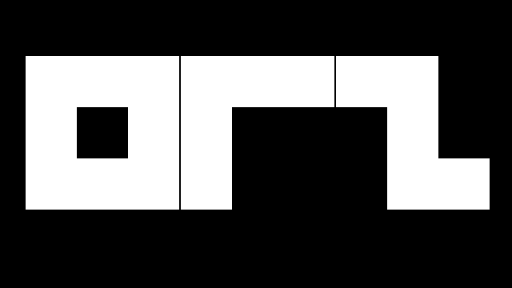The boring result, this image can be rendered with 52 bytes of raw x86 code (no API uses), doing a longer string wouldn't add much

#### What is ORZ ?

Just a funny alias i am using for my demoscene stuff, it is related to the Orz alien species of my favorite adventure game : Star Control 2

### Font / Typeface

Due to size constraint i stayed with a simple 3x3 monospaced font which seemed readable enough for the three letters i am focusing with.

A 3x3 font also has advantages when it come to packing the font into a 32-bit value (as a Bit Field) since you can pack as much as 3 letters into one value, it only use 9-bit for a single glyph.

3x3 is at the edge of being readable but it is still okay for most letters.

The typeface is thus quite simple and looks like this one:3x3 Typeface by Anders de Flon

Anyway this later got me into looking at the tiniest typeface to exist, it is a fun topic with some peoples doing readable tiny typefaces (nanofont3x4) and there is even some with 2x3 although they are barely readable at this point.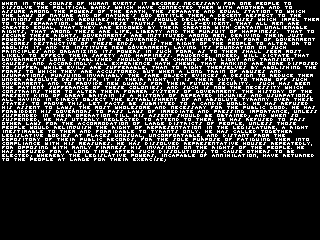nanofont3x4, one of the smallest readable typeface

There is also some 1x5? typeface which use LCD sub pixels which means that glyph are made of one pixel wide colored strips which form letters and numbers on LCD monitors. (github) Also Millitext.

Some project like Dotsies also replace letters by dots so the font become literally 1x5, goal of the project is to optimize for reading, it is unreadable unless trained for it. (here is a v2 tentative) Around 6 characters could fit into a 32 bits value, this can be quite cool to convey textual information in size limited contexts. Dotsies can be seen as the font version of Baudot code.

#### The case of stylized fonts

This article focus heavily on rendering the pretty boring typeface above but i also explored some way to render stylized fonts without much size impact by using the wire-frame renderer (see below) and adding a notion of line weight to do the fill style.

An easy way to stylize the font would be to alter the way it is rendered, doing it in perspective (bit like Star Wars scroll text) or skipping some pixels for example, many bbstro in the early 90s did that.

Another approach is to render the text somewhere and to render it again by reading it back but with modulation or compositing (fastest way to render text on DOS), most obvious would be to render it again but with an offset and dimmed, it could perhaps be applied on a per glyph basis, the renderer could perhaps be altered as well but this may be difficult for scalability.

Another approach would be to use feedback or perhaps drive it through diffusion (Laplace or Poisson equation; basis of the old-school fire effect as seen below), a cellular automaton or a chaos process or a free running Lindenmayer system.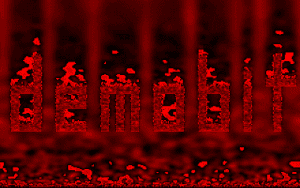Bit Hell by jendo

Many cheap decoration could also be added to beautify the logo such as a mirror effect + watery effect through distortion of the reflected image.

Doing curve rendering may be interesting although the bytes cost might be high.

Here is some stylized rendering example which can be done in ~256 bytes :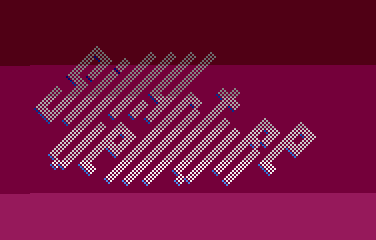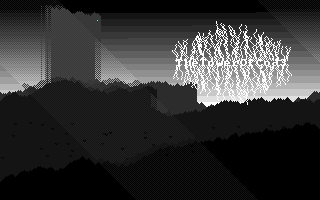rendered as pseudo 3d (cube renderer is very small !) with diffusion process on the right (also small)different kind of diffusion for a neon style (also very small !)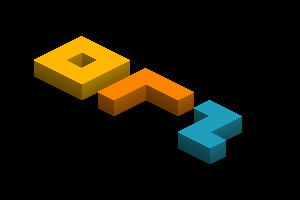slime moldLindenmayer system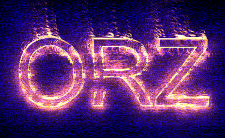easy one

### Bit field

Packing data into a bit field (e.g. using bits as an array where a bit represent a glyph pixel) was quite mandatory to save space, 9-bit is required to encode a glyph since it is a 3x3 font so ones can just use a single 32-bit value to represent 3 glyph at a time (or a 64-bit value to represent 7 glyph etc.), saving space.

Another advantage is that the data fit into a CPU register so there is no needs to access memory which may be quite heavy performances wise and also space wise.

A string could fit into a register or a set of registers, longer strings could be done with or without memory access, a stack could also be used.

Anyway, what does a string such as "ORZ" packed naively into a bit field looks like ?

#### 11-bit rows packing

`11101110110 -> 1910`
`10101000010 -> 1346`
`11101000011 -> 1859`

There is several ways to encode this, ones could let it as is and pack each row as a single 16-bit value, this waste a bit of space though.

The data could also be packed into a single 32-bit value, the issue is that the data above actually require 33 bits to be encoded (due to spaces) so there would be one glyph block left over... this can be mitigated if the missing block is actually an empty one but this is dodgy and it wouldn't suit all cases.

A better solution is to remove the space between each glyph from the bit field so a row would be 9-bit wide instead of 11, this would fit but the space between each glyph would have to be handled by the rendering code.

#### 9-bit rows packing

`111111110 -> 510`
`101100010 -> 354`
`111100011 -> 483`

The representation is less readable but it save space, now to encode this as a single 32-bit value is easy, just take the value of each rows and pack it with this formula (where << is a logical left shift and v is a bitwise disjunction) : ```(483 << 18) ∨ (354 << 9) ∨ 510```

#### Accessing data

Accessing each bit is not so different than accessing a linear 2D array and can be done with bit extraction / testing instructions or by a combination of logical shift (bit index) and bitwise AND (to extract a bit).

#### Alternatives

If one don't mind about readability there is another way to encode the data by encoding a whole glyph linearly instead of each row, this affect the rendering code. (see Renderer #2 below)

There is also variations on the packing such as row / glyph orientation in the bit field which may affect optimizations. (see Renderer #2 below)

Another way would be to use some kind of run-length encoding but this is only advantageous for some set of glyph, perhaps it would work in combination ?

#### Usage in < 256 bytes intros

The usage of bit fields is quite common in small intros as a form of data compression such as the RSI logo in Centurio 256 bytes DOS intro, the top left logo make uses of 8x8 text blocks (it calls DOS API / interrupt putch) and is packed into a single 32-bit value, the whole code for the logo is about 30 bytes :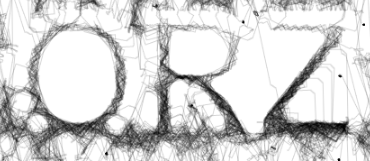Centurio, a 256 bytes DOS intro by Baudsurfer / RSI; cubes are also made of characters

Here is the whole Centurio x86 assembly text renderer (fasm syntax) :

```  mov cl,30           ; sigma of bit-glyphs a:mov eax,171445ddh   ; logo 10111000101000100010111011101b   shr eax,cl          ; bt eax,ecx;  ^reversed logo 29-bit bitmask%10   salc                ; xor al,al=ascii(NUL)~space glyph=mov al,20h   jnc c               ; cf=block (ie: non-space)   mov al,0dbh         ; block glyph c:int 29h             ; fast putch(al)   mov al,cl           ; al=cl   aam 0ah             ; =aam 10=>ah=cl/10 al=cl%10   jnz e               ; time for crlf-2*rows ?   mov w[fs:450h],ax   ; bda curs pos col=[40:50h]=cx%10 row=[40:51h]=int(cx/10) e:loop a              ; process 30 bi```

All of this is not limited to text rendering of course, bit fields can be used to encode all sort of things, boolean values (known as flags), volumetric data, tiny pixels art that you upscale on render just like a glyph so ones can make some tiny sprites or background. I'd bet that many intros are doing this for the geometry of some shapes, then they change colors (or even the representation so that it is less blocky, see the fancy fonts below) with some algorithm at runtime.

Another useful use case is also doing tiny textures by sampling the bitfield instead of using the common XOR patterns you see in small intros.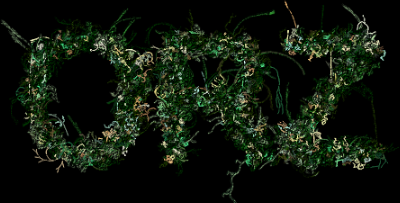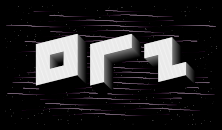lilia and sammie 256 bytes by Sensenstahl; probably not bitfield based but the "sprites" could be !neon by Sensenstahl; not really bitfield again but could be stored into a bitfield

Another idea would be to render tiles and modifying the tiles content by just modulating the bit fields or map a set of tiles differently or do simple transforms using bitwise operations such as mirroring, once you have a complete set of tile operations you can combine them to compose detailed patterns / images, ones could also animate tiles individually by switching values in a cyclic fashion (or just modifying a palette if using indexed color), this might give all sorts of cool patterns ? This is perhaps the way the impressive 256 bytes intro MEMS by Digimind works although just pure speculation. (didn't look at the code)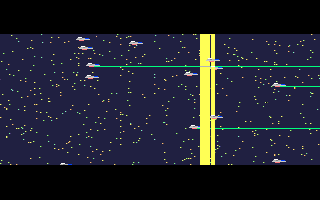MEMS, a 256 bytes DOS intro by Digimind

### Renderer : Introduction

The sections below show the working p5js code of "generic" bit field text rasterizer, there is also some C code and x86 code. They all basically draw the first image of this article. ("ORZ")

Most of the code below focus on drawing 3 glyph made of 64x64 blocks, it is trivial to extend them to render more. (if you just add a loop + put the string bit field data into an array / stack and iterate)

The output code generated by GCC vary with GCC version, compiler options (-m32 -Os -fomit-frame-pointer) and code so the binary size don't tell much (approximation) except seeing there is a potential to bring it down even more with handwritten assembly.

C code use a 32-bit pixels buffer.

The code is focused on 32-bit bit field but any size works, it will just affect some instructions and the amount of glyph that can be packed.

I also avoid floating-point arithmetic (generally not recommended for size coding) and division / multiplication instructions as much as possible, i wanted the code to be compatible with low complexity CPU, this include old CPU without div / mul instructions, i also try to fit all ideas from this context.

The text can be scaled easily.

### Renderer #1

This algorithm iterate over a whole row area and rasterize the associated bit field content.

There is 3 variations :
1. with rows as three separate values (with spaces between glyph)
2. non generic variant with single 32-bit value (a row is encoded as 11-bit values which include spaces; the last glyph block will be missed which is okay for some set of glyph)
3. with single 32-bit value (a row is encoded as 9-bit values which does not include spaces)
Modifying text height is easy but width is not so flexible as-is unless the bit index is computed differently.

The second variant avoid handling the spacing with a conditional so it is probably the smallest for this renderer although not adapted to all strings.

8 characters can be drawn as-is with the first method and 10 if you don't pack spaces.

```function setup() {   pixelDensity(1)   createCanvas(960, 540)     background(0) } function draw() {   loadPixels()   const rowHeight = 90     // row area to scan   const pstart = width * 4 * 32   const pend = pstart+(width * rowHeight)   // iterate over a whole row   for (let i = pstart; i < pend; i += 1) {     const xOffset = 128     const x = i % width - xOffset     const bi = x >> 6 // bit field index          // 1.     // string rows     const line1 = 887     const line2 = 533     const line3 = 1559     // get a row bit from given index and make it either white (256) or black (0) with << 8 (note : in C this would be a multiply because of overflowing issues, there is also a trick by using negative numbers instead of a multiply)     b1 = ((line1 >> bi) & 1) << 8     b2 = ((line2 >> bi) & 1) << 8     b3 = ((line3 >> bi) & 1) << 8          // 2. non generic variant with single 32-bit value     //    only works if you don't care about the last glyph block /*     let logo1 = 2245045111     b1 = (((logo1 & 2047) >> bi) & 1) << 8     b2 = ((((logo1 >> 11) & 2047) >> bi) & 1) << 8     b3 = ((((logo1 >> 22) & 2047) >> bi) & 1) << 8 */       // 3. generic with single 32-bit value     //    not encoding spaces /*     let logo2 = 104667903     b1 = (((logo2 & 511) >> bi) & 0x1) << 8     b2 = ((((logo2 >> 9) & 511) >> bi) & 0x1) << 8     b3 = ((((logo2 >> 18) & 511) >> bi) & 0x1) << 8 */     // first row     let index = i * 4     pixels[index + 0] = b1     pixels[index + 1] = b1     pixels[index + 2] = b1     // second row     index += width * rowHeight * 4     pixels[index + 0] = b2     pixels[index + 1] = b2     pixels[index + 2] = b2     // third row     index += width * rowHeight * 4     pixels[index + 0] = b3     pixels[index + 1] = b3     pixels[index + 2] = b3   }   updatePixels() }```

They are all about 90 bytes in C with GCC 12.2, quite small and simple:The code above draw each row separately, an additional loop can be added to avoid it, example with optimization on the third variant :

```const logo = 104667903 const rowHeight = 9 << 3 // row height is based on row iteration value (step) to optimize   const pstart = 128 * width const pend = pstart + (rowHeight * width) for (let row = 0; row <= 18; row += 9) {   const rowData = (logo >> row) & 511   for (let i = pstart; i < pend; i += 1) {     const x = i % width     if (x % 192) {       const bi = x >> 6       const br = ((rowData >> bi) & 1) * 255       const xp = 192       const yp = (row << 3) * width       const index = (i + xp + yp) * 4       pixels[index + 0] = br       pixels[index + 1] = br       pixels[index + 2] = br     }   } }```

Note how the rows spacing / glyph height is handled by re-using the row iteration value. It handle spacing between glyph although it is less flexible. (1px space)

In C it compile down to ~90 bytes of x86 code (not counting the stack push / pop for the function); ~81 bytes without space between glyph (by removing the costly conditional).

### Renderer #2

This algorithm iterate per glyph, the text is encoded per glyph row in the bit field, it can be quite efficient space wise with more optimization.

```function setup() {   pixelDensity(1)   createCanvas(960, 540)     background(0) } function draw() {   loadPixels()   // bit field data   let logo = 105684975     // text position   const offsetX = 128   const offsetY = 128   let ox = offsetX + offsetY * width     // some constants   const blockWidth = 64   const blockHeight = 64   const glyphWidth = 64 * 4   const glyphHeight = 64 * 3   // iterate until there is no glyph   while (logo > 0) {     // iterate whole glyph on screen     for (let i = 0; i < glyphWidth * glyphHeight; i += 1) {         const x = i & 255         const y = i >> 8         const bi = x >> 6         const bbi = bi + (y >> 6) * 3 // bit field bit index         const br = ((logo >> bbi) & 0x1) * 255 // get glyph bit from given index         if (bi < 3) { // spacing           const index = (ox + x + y * width) * 4           pixels[index + 0] = br           pixels[index + 1] = br           pixels[index + 2] = br         }       }       // go to the next glyph     logo >>= 9     ox += glyphWidth   }   updatePixels() }```

The conditional and x / y computation can be avoided by introducing an additional loop. ~94 bytes of x86 code generated by GCC.

A variation of this algorithm might render the glyph per column so the logo is encoded per column instead of per row, this help for optimization and avoid some computation and shrink the x86 code to ~86 bytes :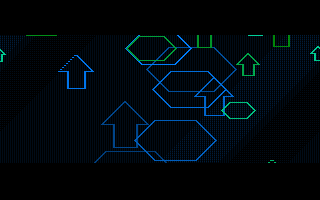The glyph loop can be split in two loop to avoid computation and the first loop can be a for loop which help to shrink it to ~83 bytes of x86 code :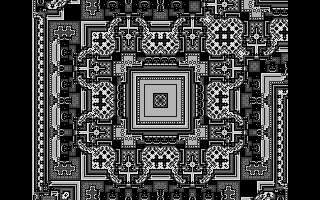This can be optimized furthermore by encoding the logo backward and using negative number as a way to avoid masking (~77 bytes) :

```int width = 960; int offsetX = 128; int offsetY = 128; int ox = offsetX + offsetY * width; unsigned int logo = 4160300832; int blockWidth = 64; int glyphHeight = 64 * 3; for (int o = ox; o < offsetX + offsetY * width + blockWidth * 11; o += blockWidth) {   for (int y = 0; y < glyphHeight; y += 1) {     int bi = y >> 6;     for (int x = 0; x < blockWidth; x += 1) {       int br = -((logo << bi) >> 31);       int index = o + x + y * width;       buffer[index] = br;     }   }     logo <<= 3;   if (!(unsigned char)o) {     o += blockWidth;   } }```

### x86 implementation

Here is a size optimized version of the C code above (adapted for 1920xN resolution), this beat GCC output:

```mov esi,4159852416 ; bit field logo mov eax,128 + 128 * width b:   mov ch,64*3-1   mov edx,esp   gh:     push 64     pop edi     bw:       mov cl,ch       shr cl,6       mov ebx,esi       sal ebx,cl       sar ebx,31       ; (o + x) * 4 + y * width       lea ebp,[edi + eax]       mov dword [edx + ebp * 4],ebx       dec edi       jns short bw     add edx,1920*4     dec ch     jnz short gh   test al,al   jne cont1     add eax,64   cont1:     add eax,64     sal esi,3 jnz short b```

63 bytes, this was my first try.

Note : esp point to the target buffer in this case.

#### Using extensions

Here is a 62 bytes version which use BMI2 (Bit Manipulation Instruction) set (shlx) to free CL register so it can be used to replace a loop with loop instruction, advantage is that it is also fast since it doesn't output black values (loopne):

```mov esi,4159852416 ; bit field logo mov ebx,128 + 128 * width b:   mov al,64*3-1   mov edx,esp   gh:     mov cl,64     bw:       mov ebp,eax       shr ebp,6       shlx edi,esi,ebp       sar edi,31       ; (o + x) * 4 + y * width       lea ebp,[ecx + ebx]       mov dword [edx + ebp * 4],edi       loopne bw     add edx,1920*4     dec al     jnz short gh   test bl,bl   jne cont1     add ebx,64   cont1:     add ebx,64     sal esi,3 jnz short b```

There is also the BEXTR instruction specifically made for extracting a bit field but didn't find it useful for my case yet.

#### Smallest "generic" so far (59 bytes)

Here is a 59 bytes version, i realized that the shifting can be replaced by a simple bit test which set the carry flag and the sbb instruction can be used to avoid a jump by setting a register to either -1 (full white) or 0 (black) depending on the carry result:

```mov esi,32657391 ; bit field logo mov ebx,128 + 128 * width b:   mov al,64*3-1   mov edx,esp   gh:     mov cl,64     bw:       mov ebp,eax       shr ebp,6       bt esi,ebp       sbb edi,edi       ; (o + x) * 4 + y * width       lea ebp,[ecx + ebx]       mov dword [edx + ebp * 4],edi       loopnz bw     add edx,1920*4     dec al     jnz short gh   test bl,bl   jne cont1     add ebx,64   cont1:   add ebx,64   sar esi,3 jnz short b```

Not really satisfied by how the spacing is handled on all the generic ones since it fail with higher start position, it is also quite costly. (7 bytes) Perhaps there is a better way ?

#### With encoded spaces (52 bytes)

A variant which encode spaces in the bit field, this simplify the rendering code, there is one missing block at the end. (which is okay for a string such as "ORZ")

```mov esi,2081583599 ; bit field logo mov ebx,150 * 4 + 100 * width*4 b:   mov al,64*3-1   mov edx,esp   gh:     mov cl,64     bw:       mov ebp,eax       shr ebp,6       bt esi,ebp       sbb edi,edi       lea ebp,[ecx + ebx]       mov dword [edx + ebp * 4],edi       loopnz bw     add edx,1920*4     dec al     jnz short gh add ebx,64 sar esi,3 jnz short b```

### Wire-frame renderer

This section is about rendering wire-frame text; glyph made of lines. A simple renderer would just take care of drawing each lines, this is efficient for few characters:

```function setup() {   pixelDensity(1);   createCanvas(1920, 768)   background(0)     loadPixels()     // start logo position   let line_length = 50 // also scale   let offx = (height / 2 - line_length / 2) * width * 4 + (width / 2 - line_length * 5 / 2) * 4     for (let i = 0; i < line_length; i += 1) {     for (let k = 0; k < 3; k += 1) { // just to handle all color components (same as * 4)       let c = 255 // color       // O       pixels[offx + (i * width) * 4 + k] = c       pixels[offx + (line_length + i * width) * 4 + k] = c       pixels[offx + i * 4 + k] = c       pixels[offx + (i + line_length * width) * 4 + k] = c       // R       pixels[offx + (line_length*2+i * width) * 4 + k] = c       pixels[offx + (line_length*2+i) * 4 + k] = c       // Z       pixels[offx + (i + line_length*4) * 4 + k] = c       pixels[offx + (i + line_length*4 + (line_length - i) * width) * 4 + k] = c       pixels[offx + (i + line_length*4 + line_length * width) * 4 + k] = c     }   }   updatePixels() }```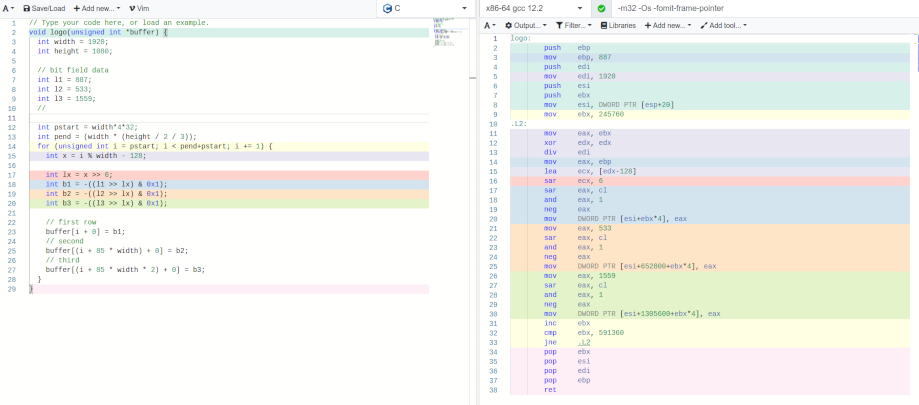Result is 79 bytes of x86 code (assume a 1920x768 buffer at esp, the text is centered):

```mov cl,150 ; line length lea eax,[esp+4725540] ; start screen offset dec esi ; assume esi was 0 before and set it to -1 (full white for a 32-bit framebuffer) plot:  imul ebx,ecx,-7676  ; O  mov dword [eax],esi  sub eax,7680  mov dword [eax+8280],esi  mov dword [esp+3573540+ecx*4],esi  mov dword [esp+4725540+ecx*4],esi  ; R  mov dword [eax+8880],esi  mov dword [esp+3574740+ecx*4],esi  ; Z  mov dword [esp+3575940+ecx*4],esi  mov dword [esp+4727940+ebx],esi  mov dword [esp+4727940+ecx*4],esi  loop plot```

Many memory access / big constants is quite costly although there is a lot of symmetry and repetition to exploit, this could be handled by swapping registers or adjusting values in a second loop to save some bytes.

Here is a 72 bytes (70 bytes with artifacts) version which group the top / bottom / vertical lines as single memory access with a loop:

```mov cl,150 ; line length lea eax,[esp+4725540] ; start screen offset dec esi ; assume esi was 0 before and set it to -1 (full white) plot:  ; Z diagonal line  imul ebx,ecx,-7676  mov dword [esp+4727940+ebx],esi  ;  xor edx,edx  push eax  lea ebx,[esp+3573540]  plot2:   mov dword [ebx+ecx*4],esi ; top lines   mov dword [eax],esi ; vertical lines   ; bottom lines   cmp edx,1   jz short skip    mov dword [ebx+1920*150*4+ecx*4],esi   skip:   add eax,150*4;can be ax (-1 byte with artifacts)   add ebx,300*4;can be bx (-1 byte with artifacts)   inc edx   jpo plot2  pop eax  sub eax,7680  loop plot```

#### Generic ?

A generic tiny wire-frame text renderer may also use a bit field which would encode 'commands' like some sort of simplified Logo language (see Turtle graphics), the data could be a 4-bit value (or 2-bit if ones don't need variable length lines) where 2-bit would be the line length and 2-bit would encode the direction and axis so ones can encode 8 lines in a single 32 bits value (16 lines if ones drop the variable length line!). Would be quite effective at rendering any types of outline and could be optimized for special cases.

There is some attempts in the demoscene of peoples doing something like this, Georgy Lomsadze which draw the logo outline progressively, it also poke into the palette so the colors rotate giving some nice retro touch, i don't know which algorithm this intro use but it seems to do well to pack a lot of lines in few bytes.

There is also Axon DOS intro by Baudsurfer which draw a stylized logo with 70 bytes of code, it use 2 bytes letter origin, 3 bits for direction and 5 bits line length for a total of 8 directions. (pi / 4)

There is also Fishy by Kuemmel, not a logo but it draw a fish using bezier curves in 256 bytes !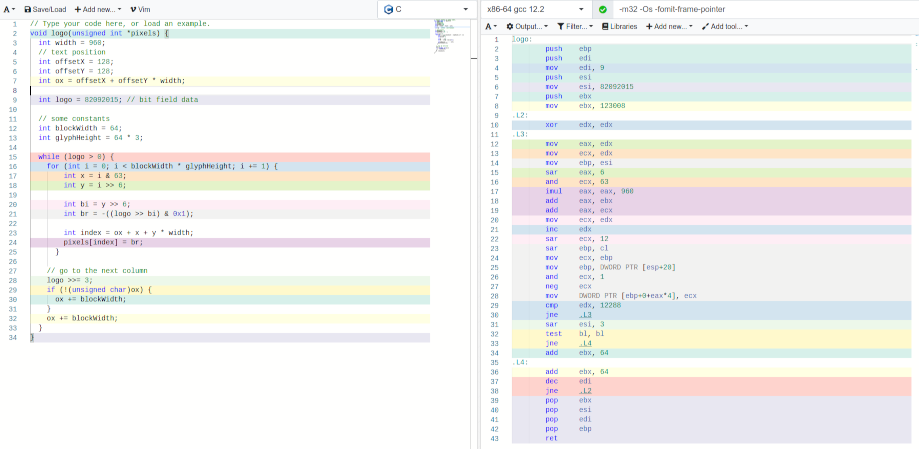256 bytes DOS intro 175 bytes of code, 79 bytes of data

with more data a stylish font could be drawn (Batman Rises Amiga OCS demo)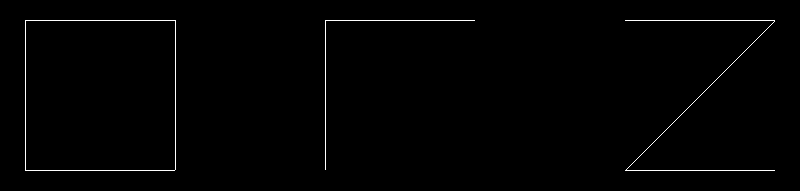256 bytes DOS intro Axon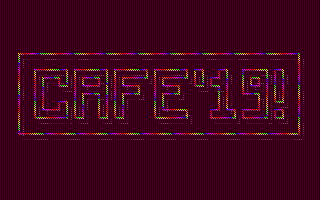256 bytes DOS intro Fishy + extra

Extending a line renderer to add more commands would be quite fun to do and would basically be a tiny implementation of LOGO, allowing complex stuff to be drawn (shapes, fractals etc.) in small code.

#### Curves ?

Bezier curves and similar algorithms could be used as seen above but i think Minsky algorithm could also be quite fitting albeit a bit more awkward to design stuff with, the base algorithm with 4 basic operators produce an ellipse and there is ways to do it in ~8 bytes on x86 by using MOVSX instruction (which result in a 8.8 fixed-point sin/cos approximation), by reusing the state simple curves can be done with 3 integers per control point with very few operations and further affine transforms could help to shape the curve.

Can also go beyond this by going piecewise by keeping track of time and modifying x,y depending on current iteration to shape the curve.

Curve approximations can be done using Fourier series which corresponds to drawing the curve using entangled ellipses.

Here is a rough prototype of doing curves with Minsky algorithm (by combining them) :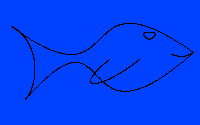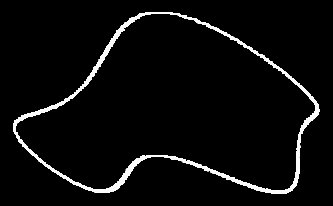first screenshot above (also added a third minsky to get roundier shape)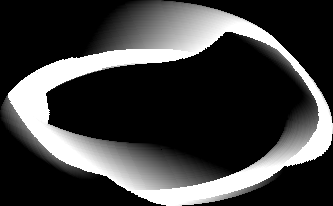can do cool Hypotrochoid figures like this (which basically relate to fourrier series; creating complex geometric patterns from the superposition of simple circular motions)

#### My version of a 'generic' wire-frame text renderer

My first version was to use a bit-field and a branch table (also called jump table), i tried with an array of pointers at first and then a simple switch statement implementation, the generated machine code was unfortunately quite dense.

First C implementation screenshot with an array of pointer, > 100 bytes of x86 code generated by GCC

Second C implementation screenshot with a switch statement, ~ 88 bytes of x86 code generated by GCC

I didn't try hand assembly on those. Also note i didn't try the C code listed here so there could be bugs, they are directly translated from working JavaScript code.

Third implementation dropped the bit-field for an array of 'cursor' index increments:

```// p5js code let lineLength = 64 let index = 0 function computeStartPosition() {   // compute start drawing position given as a precomputed index (a single instruction on x86)   let x = width / 2 - lineLength * 5 / 2 + lineLength   let y = height / 2 + lineLength / 2     // fix the pixels to the grid   x = Math.floor(x)   y = Math.floor(y)   index = (x + y * width) * 4   // } function setup() {   createCanvas(512, 512);     background(0);     computeStartPosition()     drawOnce() } function drawOnce() {   let commands = [-width, -1, width, 1, 0, -width, 1, 0, 1, width - 1, 1]   loadPixels()   for (let l = 0; l < commands.length; l += 1) {     let r = commands[l]     let c = abs(r) << 8     // draw line     for (let i = 0; i < lineLength; i += 1) {       pixels[index + 0] = c       pixels[index + 1] = c       pixels[index + 2] = c       if (r == 0) {         index += 4       } else {         index += r * 4       }     }   }   updatePixels() } function draw() { }```

The high level code is quite small and could probably be smaller with some more optimizations.

The x86 hand assembly optimized version of this code use the stack to store the index increments data which result in very small code of ~52 bytes ! It is also very flexible.

Here is the whole x86 code which draw the ORZ logo:

```; assume esi = 0, edx = 0, ecx = 1 ; prepare line drawing list (value which will be added to the index, 0 = skip drawing) mov edi, width - 1 push ecx ; 1 push edi ; width - 1 inc edi ; width mov ebx,edi neg ebx ; ebx = -width push ecx ; 1 push edx ; 0 ; zero means skip drawing but still increment with previous increment value push ecx ; 1 push ebx ; -width push edx ; 0 push ecx ; 1 push edi ; width sbb edx,edx ; edx = -1 (note: also used below as white color 0xffffffff) push edx ; push -1 push ebx ; -width ; end of lines data mov edi, 1099104 * 4 ; start position index add edi,esp ; add screen address ; at this point esi is assumed to be the drawing index position mov al,11 ; 11 lines (eax = line loop counter) cmds_loop:     pop ebx ; fetch value from the stack     mov cl,64 ; line length     line_loop:             test ebx, ebx             jne short line_draw             inc esi ; test was 0 ? skip drawing but increment index             jmp short skip_draw         line_draw:             mov dword [edi + esi * 4], edx ; draw (add computed screen/start address + drawing position)             add esi,ebx ; move drawing position, direction depend on stack fetched value         skip_draw:             loop line_loop     dec eax     jnz cmds_loop```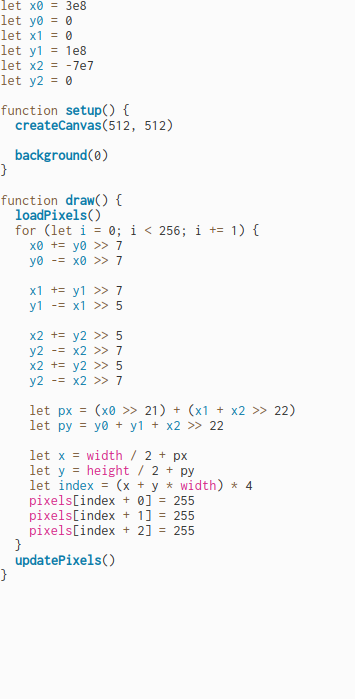~52 bytes, flexible, stack based data

The code above could be adapted to do way more and still stay small, for example adding support for variable length line is easy if ones push the line length along the increment data into the stack (ones could also pack both data as a pair of 16 bits value with values fetched by shifting the register), ones can also pack line weight for cheap stylized fonts.

The stack could be replaced by a combination of static data and perhaps lodsw instruction to fetch it but i didn't try as i felt 52 bytes was enough.

One of the disadvantage of the code above is when there is alot of line data; there is a lot of bytes wasted by the push instruction.

Thus i also tried an implementation which use a bitfield and 2-bit data (axis, direction) to draw the lines, ones could encode 16 lines as a single 32 bit value with this! It will also stay quite small with only ~59 bytes of code.

```; this use fancier x86 instruction tricks :) ; assume edi = 0 ; assume ecx = 0 for generic (it is okay here because first line is vertical) mov esi, 1099104 * 4 ; start position index add esi,esp ; add screen address mov ebp,14858637 ; bitfield logo (2-bit; axis and direction for each lines) mov bh,3 ; 3 chars chars_loop:     mov bl,4 ; 4 lines per char     cmds_loop:         sar ebp,1 ; fetch direction         sbb eax,eax ; eax = -1 if CF=0 or 0 if CF=1         or al,1 ; eax = line direction (-1 or 1)         inc ecx ; ecx = 1         sar ebp,1 ; fetch axis         mov edx,width         cmovnc ecx,edx         imul ecx ; eax = final index add: line direction * (width|1)                  stc         sbb edx,edx ; edx = 0xffffffff         ; draw line loop         push 64 ; line length         pop ecx         line_loop:             mov dword [esi + edi * 4], edx             add edi,eax             loop line_loop         dec bl         jnz cmds_loop     add edi,64 ; space (next char)     dec bh     jnz chars_loop```

Disadvantage of this code is a loss of flexibility although ones could do variable line length / diagonals by using more bits for each line. The other option is to handle them as special cases with jump / compare instructions but this only works for few letters.

Note that this code draw 4 lines for each characters so there is some wasted data for characters such as "R" by going "back and forth" on the final line, this also happen for "Z".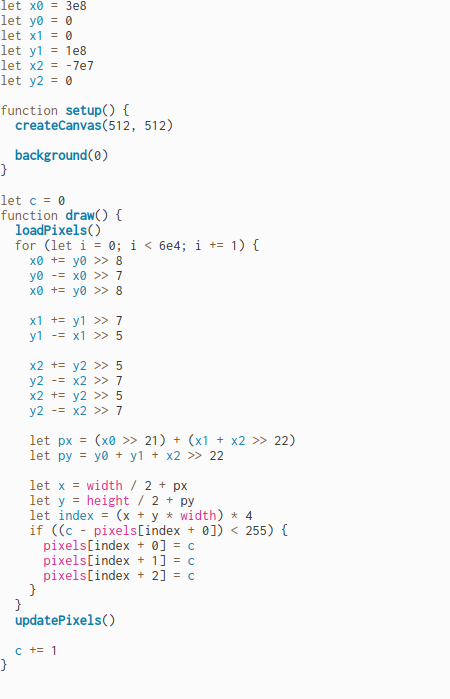~59 bytes but does not handle diagonals

I also had an idea (didn't try yet) to encode the letters by taking some intersection points and draw the associated lines, this may reduce the amount of data by exploiting the symmetry of some letters such as ones above with instruction tricks.

#### Stylized wire-frame ?

A fairly cheap idea to obtain stylized wire-frame text is to transform / project the content such as with Axonometric projection. (see above how Axon intro handle stylized text, this can serve as a base for further transform such as the one shown below)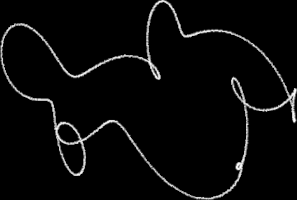projected lines

#### Blocky font and cheap fancy fonts with the wire-frame renderer ?

I realized that a blocky font could be rendered with the code above (especially the stack ones as it is very flexible) by adding a simple loop around the line drawing code and adding a notion of line weight.

Widening the lines naively produce cool glitchy blocks unless the axis is cared for, if ones don't care about perfect blocks the characters become nicely stylized with a small impact on code size.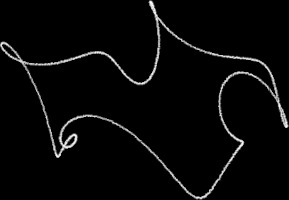adding line weight allow 'glitchy blocks' which result in stylized characters; ~70 bytes of x86 code perhaps ?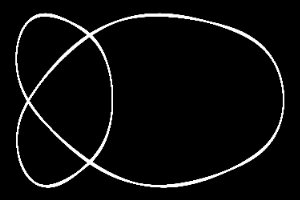alternative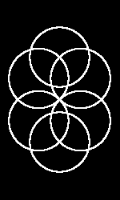glitchier version### Smaller alternative ?

Glyphs could also be rendered by taking parts of simple operations / formulas such as bitwise operators that could be assembled, would require a different renderer, i don't know how tiny it could end up but might be fun to do!

Here is a small blocky example (consider w and h are set to 64) :

```  for (let y = 0; y < h; y += 1) {     for (let x = 0; x < w; x += 1) {       let b = (x | y) >> 6 // try to replace | by + or - also try : (x + (x ^ y)) >> 7       let index = x + y * w // you can also try to rotate 45° shape (with b = x; this will produce a upside down triangle) : ((x + y) >> 1) + ((y - x) >> 1) * w       buffer[index] = b << 8     }   }```

Perhaps blocks could also be taken by taking parts of an offseted sierpinsky or munching square.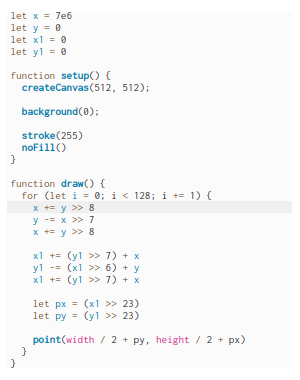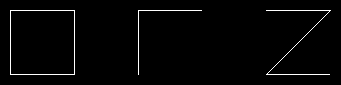result with operators : + | -

Also, an interesting approach would be to use an algorithm to generate all possible combinations of pixels (guess it work best for 2x3 font since there is 64 possibilities only), perhaps the index can be encoded efficiently with some scheme since you only want a tiny subset of characters.

### Sources

The code above are from the smallest / aggressively optimized ones but there is other type of renderer i didn't mention which may be all found here (warning: code might be a mess!), they tend to produce a bit heavier x86 code.

### Conclusion

Had some fun finding ways to do a flexible and tiny text renderer, i had several ideas for 256 bytes intros which use some letters / patterns as a seed but the fact there was no easy way to draw text with the frame buffer device (without fbcon) was kind of limiting as of now.

I also have some bare-bone OS development ideas which may use a tiny renderer like this.

Maybe some of these renderer are smaller on ARM platforms even if code density is greater since it is possible to do arithmetic + bit shifting / conditions as a single instruction.

For size coding goal ones can use a frame buffer resolution of like 1024x768 to shrink the code even more, this help doing instruction tricks / using logical operators as a size optimization.

Going for lower resolutions / bpp (why not even early monochrome ? :D) may also allow the use of more 16-bit / 8-bit registers and one byte instructions such as STOSB. This is common on DOS.

Anyway, here is the first prototype version of a 256 bytes frame buffer Linux intro i am making with that code, it is some ambigram logo which is used as a seed for feedback / automata (diffusion) stuff, quite similar code to my Lovebyte 2022 entry.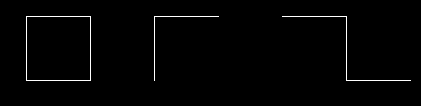Image generated by a ~186 bytes x86 program (not including fb setup/code)

back to top